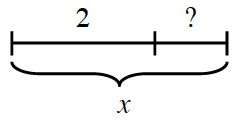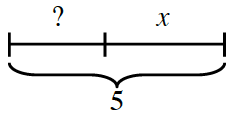### Home > INT2 > Chapter 9 > Lesson 9.4.1 > Problem9-136

9-136.

Write an expression for the unlabeled portion of the segment in each diagram.

1.We see that $x$ is the length of the entire line segment. Can we subtract something from $x$ to find the missing length?

$x-2$

1.$5-x$